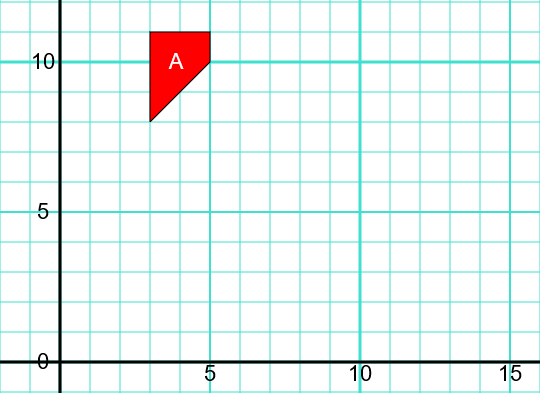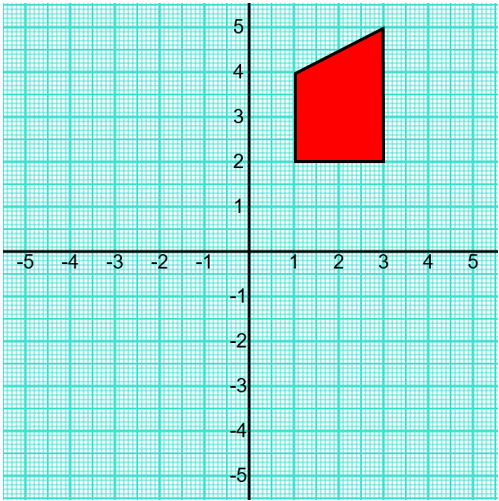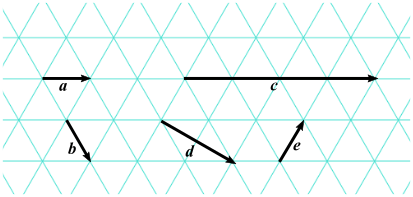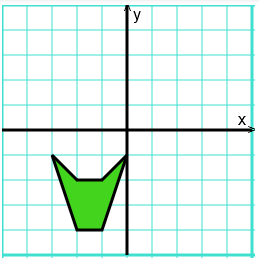# Exam-Style Questions on Transformations

## Problems on Transformations adapted from questions set in previous Mathematics exams.

### 1.

GCSE Higher

Shape A can be transformed to shape B by a reflection in the y-axis followed by a translation $${c \choose d}$$Find the value of $$c$$ and the value of $$d$$.

### 2.

GCSE Higher

The shape A is drawn on the coordinate grid as shown below.Sally and Eddie each transform the shape A onto shape B.

• Sally uses a reflection in the line y = 7 followed by a rotation of 90o anticlockwise about the point (9,9).
• Eddie transforms shape A first with a reflection in the line $$y = x$$ followed by his favourite transformation.

(a) Draw and label shape B.

(b) Describe fully Eddie's favourite transformation.

### 3.

GCSE Higher

The diagram shows a red trapezium drawn on a grid.The trapezium is subjected to two transformations, one after the other.

One transformation is a reflection in the line $$y=x$$.

The other transformation is a reflection y-axis.

### 4.

GCSE Higher

(a) Shape $$A$$ is translated to shape $$B$$ using the vector $$\begin{pmatrix}m\\n\\ \end{pmatrix}$$. What are the values of $$m$$ and $$n$$?(b) Vectors $$a, b, c, d$$ and $$e$$ are drawn on an isometric grid. Write each of the vectors $$c, d$$ and $$e$$ in terms of $$a$$ and/or $$b$$.### 5.

GCSE Higher

Plot the following points in order then join them up in order to make an irregular hexagon.

$$(-3,-1), (-2,-2), (-1,-2), (0,-1), (-1,-4), (-2,-4)$$Enlarge the hexagon by a scale factor of $$2\frac12$$, centre (-3,-4).

If you would like space on the right of the question to write out the solution try this Thinning Feature. It will collapse the text into the left half of your screen but large diagrams will remain unchanged.

The exam-style questions appearing on this site are based on those set in previous examinations (or sample assessment papers for future examinations) by the major examination boards. The wording, diagrams and figures used in these questions have been changed from the originals so that students can have fresh, relevant problem solving practice even if they have previously worked through the related exam paper.

The solutions to the questions on this website are only available to those who have a Transum Subscription.

Exam-Style Questions Main Page

Search for exam-style questions containing a particular word or phrase:

To search the entire Transum website use the search box in the grey area below.